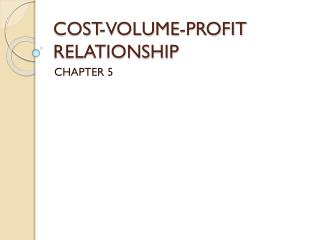# COST-VOLUME-PROFIT RELATIONSHIP - PowerPoint PPT PresentationDownload PresentationCOST-VOLUME-PROFIT RELATIONSHIP

COST-VOLUME-PROFIT RELATIONSHIP
Download Presentation## COST-VOLUME-PROFIT RELATIONSHIP

- - - - - - - - - - - - - - - - - - - - - - - - - - - E N D - - - - - - - - - - - - - - - - - - - - - - - - - - -
##### Presentation Transcript

1. COST-VOLUME-PROFIT RELATIONSHIP CHAPTER 5

2. CVP Formula • Sx = VCx + FC + P • S= Selling Price • X= Sales Volume • VC = Variable Cost per unit • FC = Fixed Cost • P= Profit • Very powerful equation • If all else fails just work the equation

3. Things you can find out using CVP formula • Breakeven points • Units to sell to get a certain profit • How many more to sell if Fixed Cost increased • Selling Price

4. Apply CVP Formula • Selling Price \$36 • Variable Cost \$24 per unit • Fixed Costs \$12,000 • Units 2,000 • Profit= ? • Put in CVP formula

5. CONTRIBUTION MARGIN • The amount that contributes to fixed costs and profits i.e Contribution • Calculated In per unit, \$ and in % • \$100 Sales • 60 VC • \$ 40 CM 40% Ratio (\$40/\$100= .40) • 35 FC • \$ 5 NI

6. CONTRIBUTION MARGIN FORMAT Income Statement • SALES • -VARIABLE COST • =CONTRIBUTION MARGIN • - FIXED EXPENSES • NET OPERATING INCOME • Exercise 5-1 page 213

7. Application of CVP Data • Exercise 5-5 page 214 • 1- Increase advertising budget • 2- Increase quality of product

8. BREAK EVEN (BE) IN UNITS & \$ • The units or \$ that will cover the fixed costs with no profit. • Sx – VCx= FC BE in equation method • FC/CM% = BE\$ CM Method • You can determine: BE in units, BE in \$ • Exercise 5-7 pg 214

9. PROFIT PLANNING • Answers these questions: • How many do I need to sell to make \$100,000 profit • For example: If I reduce my fixed costs by \$2,000 and increase my sales in units by 100 how will my profit change?

10. Target Profit Analysis • Formula for units to make a \$ profit • FC + Profit • Unit CM • X sales price = Sales to attain target profit • Exercise 5-6 pg 214 • 1- equation method • 2- CM approach

11. Margin of Safety (MS) • Amount you can drop before losses are incurred • How much can our sales drop before we start losing money • Every company has a different % because each is structured differently • How much excess you have over break even. • How much you have after you cover your fixed costs.

12. Margin of Safety formula • Budgeted Sales – BE\$ = MS\$ • MS\$/Budgeted Sales=MS% • Example: • Sales \$100,000 • BE\$ 87,500 • MS\$ \$ 12,500 / 100,000 = 12.5% • Exercise 5-8 page 214

13. Operating Leverage (OL) pg 202 • How sensitive income is to a % change in Sales \$ • How a % change in Sales volume will affect profits. • It is a Multiplier • If OL is high a small % change in Sales will reuslt in a higher change in NI

14. Operating Leverage Formula • Contribution Margin \$ • Net Income in \$ • It OL is 2 and sales increased by 5% then net income will increase by 10% • Exercise 5-9 pg 215

15. Operating leverage proof • Sales 100,000 110,000 • VC 60,00066,000 • CM 40,000 44,000 • FC 35,000 35,000 • NI 5,000 9,000 \$4,000 • OL 40,000/5000= 8 times x 10% • 80% x \$5000 = \$4000

16. CM Ratio • Another way to determine effect on net income • Change in Net Income with the change in Total Sales • If we sell 10,000 more units, how would our net income increase? • 10,000 X25%CM= 2500 change in units X \$24 per unit = \$60,000 increase in NI • How much would our net income increase if our sales increase by \$240,000 • \$240,000 X 25% = \$60,000

17. Sales Mix Multi Product CM • Proportions in which a company’s products are sold • Mix that will yield the greatest profit • Steps to determine • 1- Total all sales • 2- VC % for each product and total sales • 3- = CM% for all sales • 4- Determine total BE\$ FC/CM% • 5- Each product % of total sales X BE\$ • 6- Use VC% for each product for VC • 7- =CM for each product = total fixed costs • Page 206 Exhibit 5-4 • Exercise 5-10, pg 215